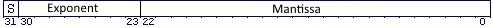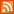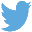Bucaro TecHelpHTTPS Encryption not required because no account numbers orpersonal information is ever requested or accepted by this siteCustom SearchBinary Floating-Point Numbers

A computer with n-bit word size is capable of handling unsigned integers in the range of 0 to 2n - 1 in a single word. For a 32-bit computer this would be numbers up to 4,294,967,295. Floating-point numbers allow you to use the very large, and very small, numbers commonly found in scientific calculations. In fact, sometimes they're called "scientific notation".

A floating-point number has two parts, the number part and the radix. For example the mass of the sun is 1.989x1030 kg. The diameter of a red blood cell is 3×10-4 inches. The 1.989 and the 3 are the number parts, the 1030 and the 10-4 are the radix part.

Note, some displays aren't capable of displaying superscripts, so they use a capital E to indicate the following number is an exponent. For example the mass of the sun can be expressed as 1.989E30 kg. The diameter of a red blood cell can be expressed as 3×E-4 inches. Or a limited display might express it as 3 × 10-4, leaving out the superscript and the E.A binary floating-point number consists of three parts, the sign bit, the mantissa and the exponent. A sign bit of 1 indicates a negative number. A sign bit of 0 indicates a positive number. In a 32-bit system, the exponent is 8 bits following the sign bit, and the number part is 23 bits.

In a 64-bit system, the exponent is 11 bits and the mantissa is 53 bits. A 64-bit floating-point number is called double-precision as opposed to 32-bit being referred to as single-precision. The mantissa is now called the significand because it's size controls the accuracy of the number.

Normalizing a Binary Floating-Point Number

Before a binary floating-point number can be correctly stored, it must be normalized. Normalizing means moving the decimal point so that only one digit appears to the left of the decimal point. This creates the mantissa. The exponent then becomes the number of positions the decimal point was moved. If the decimal point was moved left it creates a positive exponent. If the decimal point was moved right, it creates a negative exponent.

For example, the floating-point binary number 1101.101 is normalized by moving the decimal point 3 places to the left. The mantissa then becomes 1.101101 and the exponent becomes 3, creating the normalized floating point binary number 1.101101x23.

Biasing a Binary Floating-Point Number

We could store negative exponents as two's complement binary numbers, however this would make it more difficult (programmatically) to make number comparisons (< == >). For this reason a biasing constant is added to the exponent to make sure it's always positive.

The value of the biasing constant depends upon the number of bits available for the exponent. For a 32-bit system, the bias is 12710, which is 01111111 binary. So for example;

If exponent is 5, biased exponent is 5 + 127 = 13210 = 10000100 binary.

If exponent is -5, biased exponent is -5 + 127 = 12210 = 01111010 binary.

Although exponent biasing makes number comparisons faster and easier, there are always trade-offs. The actual exponent is found by subtracting the bias from the stored exponent. This means the largest exponent possible for a 32-bit system will be -12710 to +12810.

If you can't fit your number within that range you need to use a double-precision binary floating-point number. A double-precision number is biased by adding 1023 by adding 102310 for an exponent in the range -102210 to +102310.

 .menubtn { margin-top:10px; margin-left:50px; width:220px; opacity:1; border-style:solid; border-color:#009000; background-color:#66ff66; transition-property: opacity; transition-duration: 1s; transition-timing-function: ease-out; } .menubtn:hover { opacity:1; background-color:#e5ff23; } Menu - MoreComputer ArchitectureRSS FeedFollow @Stephen Bucaro[Site User Agreement] [Privacy Policy] [Site map] [Search This Site] [Contact Form]
Copyright©2001-2019 Bucaro TecHelp 13771 N Fountain Hills Blvd Suite 114-248 Fountain Hills, AZ 85268